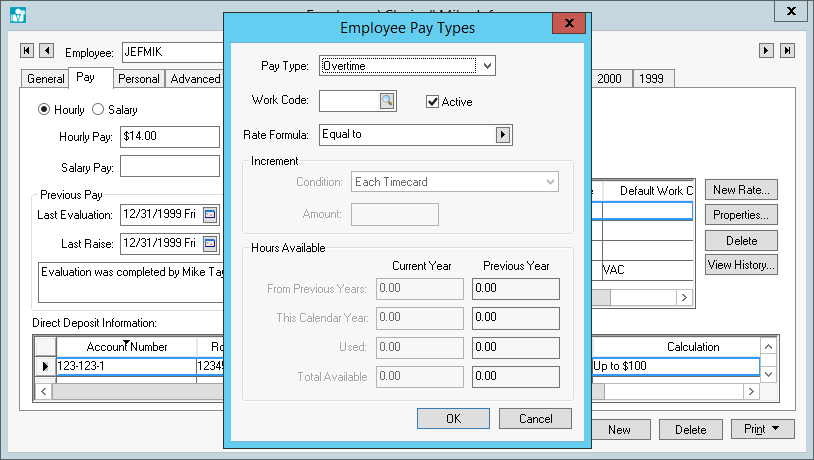# Overtime, Double, and other Calculated Pay Rates

The EBMS software allows the user to set pay rates based on a formula rather than a fixed pay rate. The most common pay rate that is based on a formula is the overtime rate. Overtime pay is normally calculated by adding 50% to the average pay rate as shown below:Review the Getting Started > Pay Types section for instructions on how to create a Pay Type such as Overtime if it does not already exist.

Open an employee record and click on the Pay tab as shown above. Select a formula based Pay Type and click on the Properties button to review the Rate Formula.

Formula rates are calculated from the average standard pay rate rather than calculating from the base rate. Note that the overtime rate (or other formula rate) may not equal the rate shown on the Pay tab if the average rate of the standard pay varies from the employee's hourly rate. The average standard pay may vary if the user adjusts individual pay rates within the timecard or if the pay varies based on prevailing wages etc. Review the Processing Payroll > Calculate Overtime section for more details.